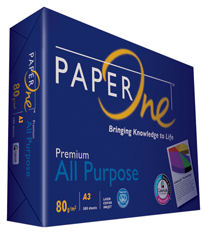Search IntMath
Close

450+ Math Lessons written by Math Professors and Teachers

5 Million+ Students Helped Each Year

1200+ Articles Written by Math Educators and Enthusiasts

Simplifying and Teaching Math for Over 23 Years

# Paper math

By Murray Bourne, 09 Jun 2009I bought a ream of paper today. This is actually fairly rare because we recycle paper as much as possible. A standard ream is 500 sheets.

It brought to mind a question I used to give my trainee elementary school teachers. I asked them to estimate the weight of a ream of A4 paper, assuming the commonly-used density of 80 g/m2 (also written as "gsm"). Estimation is a key skill in mathematics and I believe teachers should develop such a skill.

I expected the howls of protest that ensued ("We don't have enough information!" and "It's too hard!"), but after a while they settled to the task.

I'll let you think about the question while I tell you about China's voracious paper appetite.

## China's Paper Use

China currently consumes around 80 million tonnes of paper each year. This represents about 50kg per capita (per person) per year, compared to America's 280 kg per capita and Europe's 161 kg per capita.

Computers were supposed to reduce our use of paper, but of course, they have had the opposite effect. As China rapidly develops its technology and as the number of people working in middle class jobs grows, the amount of paper use will go through the roof. The environmental impact on rainforests in SE Asia will be immense.

Enjoy breathing for now...

## The weight of a ream of paper

Back to the weight of a ream of paper.

The estimation process goes something like this. A piece of A4 paper has dimensions roughly 20 cm × 30 cm, giving an area of 600 cm2.

One square meter is 100 cm × 100 cm = 10,000 cm2. The number of pieces of paper needed to cover 1 m2 is around 10,000/600 ≈ 17 pieces.

Those 17 pieces weigh 80 g, since the paper density is 80 g/m2.

The total area of the ream of paper is 500/17 ≈ 30 m2.

So the total weight of the ream of paper is 30 × 80 = 2,400 g = 2.4 kg.

Now is that question impossible - even without a calculator?

## Did you know?

Paper sizes are based on the A0 size, which has an area of 1 m2. Its dimensions are 841 cm × 1189 cm, and its aspect ratio is 1:√2. That is, the height divided by the width is √2.

To get the next size down, we just cut A0 in half giving A1 size, at 0.5 m2. This also has an aspect ratio of 1:√2 (in fact, all paper sizes have this ratio — this was deliberate so all paper sizes are a neat multiple of each other.) We keep going, halving each time, so we get A2, A3 and then A4, the standard size used in office printers. This size is exactly 1/16 of a square meter (10000/16 = 625 cm2, so our estimate of 600 cm2 was not bad.)

## Finally

BTW - The Indonesian manufacturers of the paper I bought today claim that it is made from 100% plantation fiber (for which which they ripped down rainforest) and is chlorine free. It's supposed to last "for 200 years".

Let's hope whoever is reading my pieces of paper in 200 years enjoys it.

Be the first to comment below.

### Comment Preview

HTML: You can use simple tags like <b>, <a href="...">, etc.

To enter math, you can can either:

1. Use simple calculator-like input in the following format (surround your math in backticks, or qq on tablet or phone):
a^2 = sqrt(b^2 + c^2)
(See more on ASCIIMath syntax); or
2. Use simple LaTeX in the following format. Surround your math with $$ and $$.
$$\int g dx = \sqrt{\frac{a}{b}}$$
(This is standard simple LaTeX.)

NOTE: You can mix both types of math entry in your comment.